• 对比学习代码
千次阅读
2022-01-13 18:16:03

对比学习是一种通过对比正反两个例子来学习表征的自监督学习方法。对于自监督对比学习，下一个等式是对比损失：

L i , j = − log ⁡ e x p ( z i ⋅ z j / τ ) ∑ k = 1 , k ≠ i 2 N e x p ( z i ⋅ z k / τ ) \mathcal{L}_{i,j} = - \log \frac{exp(\textbf{z}_i \cdot \textbf{z}_j / \tau)}{\sum_{k=1,k\neq i}^{2N}exp(\textbf{z}_i \cdot \textbf{z}_k / \tau)}

在很多情况下，对比学习只需要对每一个样本生成一个正样本，同一个batch内的其他样本作为负样本，实现如下：

def contrastive_loss(x, x_aug, T):
"""
:param x: the hidden vectors of original data
:param x_aug: the positive vector of the auged data
:param T: temperature
:return: loss
"""
batch_size, _ = x.size()
x_abs = x.norm(dim=1)
x_aug_abs = x_aug.norm(dim=1)

sim_matrix = torch.einsum('ik,jk->ij', x, x_aug) / torch.einsum('i,j->ij', x_abs, x_aug_abs)
sim_matrix = torch.exp(sim_matrix / T)
pos_sim = sim_matrix[range(batch_size), range(batch_size)]
loss = pos_sim / (sim_matrix.sum(dim=1) - pos_sim)
loss = - torch.log(loss).mean()
return loss


如果要用生成的负样本进行对比，代码如下：

def info_nce_loss(self, features):
labels = torch.cat([torch.arange(self.args.batch_size) for i in range(self.args.n_views)], dim=0)
labels = (labels.unsqueeze(0) == labels.unsqueeze(1)).float()
labels = labels.to(self.args.device)

features = F.normalize(features, dim=1)

similarity_matrix = torch.matmul(features, features.T)
# assert similarity_matrix.shape == (
#     self.args.n_views * self.args.batch_size, self.args.n_views * self.args.batch_size)
# assert similarity_matrix.shape == labels.shape

# discard the main diagonal from both: labels and similarities matrix
mask = torch.eye(labels.shape, dtype=torch.bool).to(self.args.device)
labels = labels[~mask].view(labels.shape, -1)
similarity_matrix = similarity_matrix[~mask].view(similarity_matrix.shape, -1)
# assert similarity_matrix.shape == labels.shape

# select and combine multiple positives
positives = similarity_matrix[labels.bool()].view(labels.shape, -1)

# select only the negatives the negatives
negatives = similarity_matrix[~labels.bool()].view(similarity_matrix.shape, -1)

logits = torch.cat([positives, negatives], dim=1)
labels = torch.zeros(logits.shape, dtype=torch.long).to(self.args.device)

logits = logits / self.args.temperature
return logits, labels

self.criterion = torch.nn.CrossEntropyLoss()
loss = self.criterion(logits, labels)


更多内容访问 omegaxyz.com
网站所有代码采用Apache 2.0授权
网站文章采用知识共享许可协议BY-NC-SA4.0授权
© 2022 • OmegaXYZ-版权所有 转载请注明出处python pytorch
更多相关内容
• 对比学习代码整理、超参数调优、日志记录结果、新数据集ohsumed
• SupContrast：监督式对比学习 此库使用CIFAR作为说明性示例，涵盖了PyTorch中以下论文的参考实现： （1）监督式对比学习。（2）视觉表示对比学习的简单框架。 损失函数 损耗函数在losses.py花费features （L2归一化...Python
• 在NeuraIPS上对比学习（Contrastive Learning）...本文为大家奉上NeurIPS 2020必读的七篇对比学习相关论文——对抗自监督对比学习、局部对比学习、难样本对比学习、多标签对比预测编码、自步对比学习、有监督对比学习
• 所以 这次就想着对比学习能不能用来解决这个问题呢 。？看了一圈，感觉simsiam是对比学习里比较简单的一种方法，好像效果也不错。 所以来看一看这个东西是怎么玩的。 simsaim 是对比学习很新的文章了。 他的训练...

目录

1 ： 事先准备 。

2 ： 代码阅读。

2.1: 数据读取

2.2： 模型载入

3 训练过程：

4 测试过程：

5 ：线性验证

6 ： 用自己数据集进行对比学习。

第一：  改数据集 ：

2 改变batch_size和图片大小。

写在前面的话 CSDN真的是'sb'中的'sb'软件， 辛辛苦苦写半天  我复制个东西过来 他就把前面的刷没了 还要我重头写？？？？？？？？？？？？神经并b

------------------------------------------------------------------------------------------------------------------------------

2022李宏毅作业HW3 是食物的分类 ，但是我怎么尝试 再监督学习的模式下 准确率都达不到百分之60 .。半监督也感觉效果不明显。 所以 这次就想着对比学习能不能用来解决这个问题呢 。？看了一圈，感觉simsiam是对比学习里比较简单的一种方法，好像效果也不错。 所以来看一看这个东西是怎么玩的。

simsaim 是对比学习很新的文章了。 他的训练方式简单来说就是 ，一张图片 ，用不同的方式去增广后形成图片对 。 然后用一张去预测另一张。 不懂得可以看朱老师的视频。

对比学习论文综述【论文精读】_哔哩哔哩_bilibili

# 1 ： 事先准备 。

代码地址 ： 好像不是官方的

下载解压。

直接在main函数的 运行 编辑配置中输入

--data_dir ../Data/ --log_dir ../logs/ -c configs/simsiam_cifar.yaml --ckpt_dir ~/.cache/ --hide_progress --download

然后在main函数加入代码：表示使用0号显卡进行训练。

os.environ['CUDA_VISIBLE_DEVICES']='0'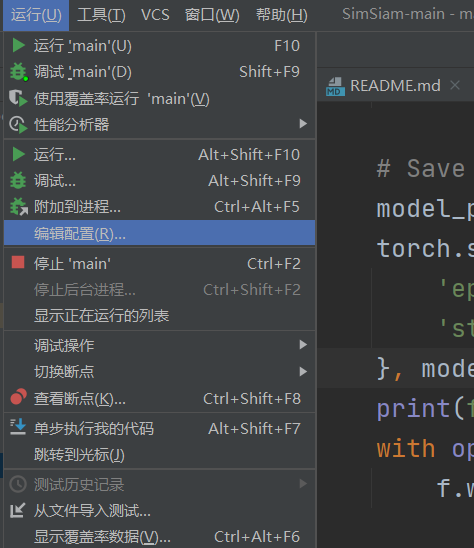# 2 ： 代码阅读。

神经网络的一个基本的框架就是 ： 数据读取 ， 模型载入， 训练，测试。 我们接下来根据这四块来看。

## 2.1: 数据读取

运行main文件 。

    main(device=args.device, args=args)

进入main 函数 。

是三个数据集的读取。

train_loader ，， memory_loader 和 test_loader。 train和memory 都是训练集的数据 他们的不同之处在于， 数据增广的方式不同。 train的增广是用来训练的 memory和test的增广都是用来测试的。由于在对比学习里，  数据增广是很重要的 ，所以这里看下数据增广的方式。
        dataset=get_dataset(
transform=get_aug(train=True, **args.aug_kwargs),
train=True,
**args.dataset_kwargs),
**args.aug_kwargs 里规定了图片大小是32. 以及这次用的是simsaim。

这里两个train  。 只有训练集的第一个train是true。 而训练集的增广方式如下

class SimSiamTransform():
def __init__(self, image_size, mean_std=imagenet_mean_std):
image_size = 224 if image_size is None else image_size # by default simsiam use image size 224
p_blur = 0.5 if image_size > 32 else 0 # exclude cifar
# the paper didn't specify this, feel free to change this value
# I use the setting from simclr which is 50% chance applying the gaussian blur
# the 32 is prepared for cifar training where they disabled gaussian blur
self.transform = T.Compose([
T.RandomResizedCrop(image_size, scale=(0.2, 1.0)),
T.RandomHorizontalFlip(),
T.RandomApply([T.ColorJitter(0.4,0.4,0.4,0.1)], p=0.8),
T.RandomGrayscale(p=0.2),
T.RandomApply([T.GaussianBlur(kernel_size=image_size//20*2+1, sigma=(0.1, 2.0))], p=p_blur),
T.ToTensor(),
T.Normalize(*mean_std)
])
def __call__(self, x):
x1 = self.transform(x)
x2 = self.transform(x)
return x1, x2 

这里依次是 ： 随机resize 然后剪切为输入大小，  也就是会随机取图片里的一块。

随机水平变换

0.8的概率调节亮度对比度和饱和度。

0.2概率灰度化

对于32的照片 不做高斯模糊。

转化为张量并标准化。

然后 对于一个输入  这里会做两次transform  call可以让这个类像函数那样被调用。

对于 测试用的训练集 。也就是memory 是下面的增广方式。  而test也是下面的增广方式 。

        else:
self.transform = transforms.Compose([
transforms.Resize(int(image_size*(8/7)), interpolation=Image.BICUBIC), # 224 -> 256
transforms.CenterCrop(image_size),
transforms.ToTensor(),
transforms.Normalize(*normalize)
])

如果输入是 224 就 先放大到256，然后中心裁剪224，之后标准化。

如果输入是32 就放大到36 再中心裁剪32 .后标准化。

用的是cifar10的数据。 其实也就相当于很普通的 读图片  然后增广， 加标签。

我们只要看getitem取出来的数据是什么就好 。

        img, target = self.data[index], self.targets[index]

# doing this so that it is consistent with all other datasets
# to return a PIL Image
img = Image.fromarray(img)

if self.transform is not None:
img = self.transform(img)

if self.target_transform is not None:
target = self.target_transform(target)

return img, target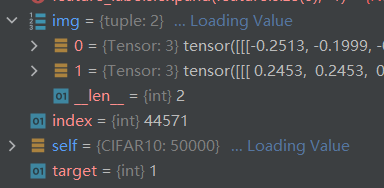注意  如果是训练集 在trans时会返回两张图片 ，所以返回的是一个元组。 而测试时 ，img就是单独的一张图片。 target也就是标签。

总结：数据部分 我们需要做一个数据集， 然后训练集的增广要返回两个结果。  当读取数据时，返回的是图片数据和标签数据。

# 2.2： 模型载入

这一部分我们来看模型 ，我们可以根据下面的伪代码来看模型长什么样子。 伪代码非常容易看懂。 aug就是增广嘛。 f来提特征，然后两个预测。 算loss 回传。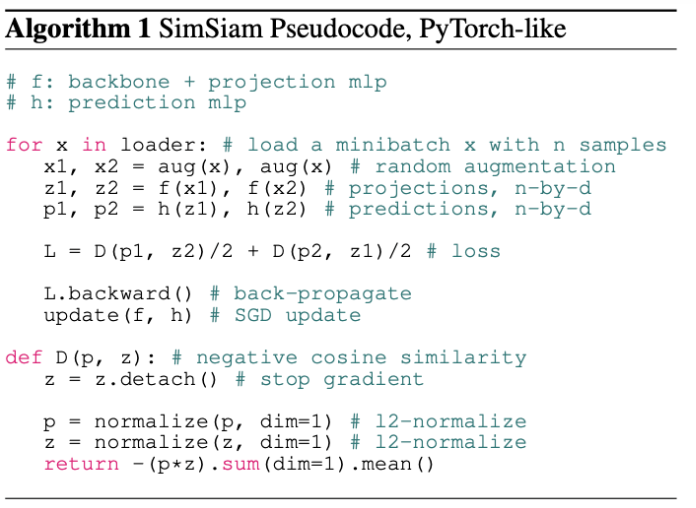model = get_model(args.model).to(device)

这句来获得模型。

    if model_cfg.name == 'simsiam':
model =  SimSiam(get_backbone(model_cfg.backbone))

backbone就是普通的res18  这里不需要预训练的模型 只需要初始模型 。

class SimSiam(nn.Module):
def __init__(self, backbone=resnet50()):
super().__init__()

self.backbone = backbone
self.projector = projection_MLP(backbone.output_dim)

self.encoder = nn.Sequential( # f encoder
self.backbone,
self.projector
)
self.predictor = prediction_MLP()

def forward(self, x1, x2):

f, h = self.encoder, self.predictor
z1, z2 = f(x1), f(x2)
p1, p2 = h(z1), h(z2)
L = D(p1, z2) / 2 + D(p2, z1) / 2
return {'loss': L}


这个就是simsam的模型了 。 projector 是个三层的普通mlp 。 encoder 就是伪代码里的f了 而predictor就是伪代码里的h了 。 我们具体来看下loss 。

def D(p, z, version='simplified'): # negative cosine similarity
if version == 'original':
z = z.detach() # stop gradient
p = F.normalize(p, dim=1) # l2-normalize
z = F.normalize(z, dim=1) # l2-normalize
return -(p*z).sum(dim=1).mean()

elif version == 'simplified':# same thing, much faster. Scroll down, speed test in __main__
return - F.cosine_similarity(p, z.detach(), dim=-1).mean()
else:
raise Exception

传说 simsaim的精髓就在于这个loss， 在于这个z.detach 也就是传说中的stop gradiant。 有了这个梯度停止， simsaim才能够训练的起来。 这时的simsaim就和k-means算法有点类似了。 说法很多 大家可以搜搜看。

其实我们可以看出来一点东西，在算loss时， p是预测值， z是标签，如果标签也要算梯度，两边就都在变了，参考我们平时的label都是不变的，确实z也不应该算梯度。

x = 2
y = 2**2
z = y+x

y.detach()
z.grad = 1

本来y是x的平方 求导等于4 所以z对x求导是5  然后不算y的梯度了 那么就只剩1了 。

这就是模型的全部了 ，输入两张图片 ，然后抽特征  预测 分别算loss

# 3 训练过程：

训练是非常普通的训练。

        for idx, ((images1, images2), labels) in tqdm(enumerate(local_progress)):

data_dict = model.forward(images1.to(device, non_blocking=True), images2.to(device, non_blocking=True))
loss = data_dict['loss'].mean() # ddp
loss.backward()
optimizer.step()
lr_scheduler.step()
data_dict.update({'lr':lr_scheduler.get_lr()})

local_progress.set_postfix(data_dict)
# logger.update_scalers(data_dict)

从测试集中抽loader  注意抽出的是两张图片 由不同transformers形成的。 之后过模型得到loss，梯度回传。 这里日志一直报错 我直接屏蔽了。

# 4 测试过程：

测试过程比较的关键。

这里是用knn算法进行测试的 ，关于knn 可以看 深入浅出KNN算法（一） KNN算法原理 - zzzzMing - 博客园

简单的说， 就是从众。 在一个大平面上有很多的点， 然后你就看离自己最近的k个点，他们的标签是啥， 然后选最多的那个当自己的标签。

            accuracy = knn_monitor(model.module.backbone, memory_loader, test_loader, device, k=min(args.train.knn_k, len(memory_loader.dataset)), hide_progress=args.hide_progress) 
def knn_monitor(net, memory_data_loader, test_data_loader, epoch, k=200, t=0.1, hide_progress=False):
net.eval()
total_top1, total_top5, total_num, feature_bank = 0.0, 0.0, 0, []
# generate feature bank
for data, target in tqdm(memory_data_loader, desc='Feature extracting', leave=False, disable=hide_progress):
feature = net(data.cuda(non_blocking=True))
feature = F.normalize(feature, dim=1)
feature_bank.append(feature)
# [D, N]
feature_bank = torch.cat(feature_bank, dim=0).t().contiguous()
# [N]
feature_labels = torch.tensor(memory_data_loader.dataset.targets, device=feature_bank.device)
# loop test data to predict the label by weighted knn search
test_bar = tqdm(test_data_loader, desc='kNN', disable=hide_progress)
for data, target in test_bar:
data, target = data.cuda(non_blocking=True), target.cuda(non_blocking=True)
feature = net(data)
feature = F.normalize(feature, dim=1)

pred_labels = knn_predict(feature, feature_bank, feature_labels, classes, k, t)

total_num += data.size(0)
total_top1 += (pred_labels[:, 0] == target).float().sum().item()
test_bar.set_postfix({'Accuracy':total_top1 / total_num * 100})
return total_top1 / total_num * 100

注意 这里的net  只是backbone  也就是resnet 而 memory 就是训练数据 不过增广方式不一样 。还有训练数据 和k值 取200.

    net.eval()
total_top1, total_top5, total_num, feature_bank = 0.0, 0.0, 0, []

一些初始化和获取类别数。

    with torch.no_grad():
# generate feature bank
for data, target in tqdm(memory_data_loader, desc='Feature extracting', leave=False, disable=hide_progress):
feature = net(data.cuda(non_blocking=True))
feature = F.normalize(feature, dim=1)
feature_bank.append(feature)
# [D, N]
feature_bank = torch.cat(feature_bank, dim=0).t().contiguous()
feature_labels = torch.tensor(memory_data_loader.dataset.targets, device=feature_bank.device)

获取大平面上的点。 从训练集抽数据， 然后获取他们的特征。

最后的feature_bank大小是49664*512 也就是将近50000条数据 每个数据都有512 维的特征。 然后做了一个转置。

        for data, target in test_bar:
data, target = data.cuda(non_blocking=True), target.cuda(non_blocking=True)
feature = net(data)
feature = F.normalize(feature, dim=1)

抽取测试集的特征。

            pred_labels = knn_predict(feature, feature_bank, feature_labels, classes, k, t)
def knn_predict(feature, feature_bank, feature_labels, classes, knn_k, knn_t):
# compute cos similarity between each feature vector and feature bank ---> [B, N]
sim_matrix = torch.mm(feature, feature_bank)
# [B, K]
sim_weight, sim_indices = sim_matrix.topk(k=knn_k, dim=-1)        #求出最大的knn_k个值
# [B, K]
sim_labels = torch.gather(feature_labels.expand(feature.size(0), -1), dim=-1, index=sim_indices)
sim_weight = (sim_weight / knn_t).exp()

# counts for each class
one_hot_label = torch.zeros(feature.size(0) * knn_k, classes, device=sim_labels.device)
# [B*K, C]
one_hot_label = one_hot_label.scatter(dim=-1, index=sim_labels.view(-1, 1), value=1.0)
# weighted score ---> [B, C]
pred_scores = torch.sum(one_hot_label.view(feature.size(0), -1, classes) * sim_weight.unsqueeze(dim=-1), dim=1)

pred_labels = pred_scores.argsort(dim=-1, descending=True)
return pred_labels


我们来看 knn是如果计算相似度的 ，也就是距离的。torch.mm表示矩阵的乘法。 我举个例子。

下面只是例子 ，真实数据需要归一化

a = [[1,2,3],
[4,5,6]]
b = [[1,2,3],
[2,4,6],
[3,6,9],
[4,8,1]]

a有2个样本， b有4个样本。 他们的特征都是3维。 现在求a   和b中哪些样本最相似。

就要让a和b中每一个样本点乘 得到 14, 28, 42, 23。数越大表示越相似，也就越近。 所以我们让a和b的转置相乘，得到：

tensor([[14, 28, 42, 23],
[32, 64, 96, 62]])

我们发现第一排 就是a的相似度， 每一列都是与b中样本的点乘结果。

    sim_matrix = torch.mm(feature, feature_bank)

所以这里的sim_matrix 就是一个512 * 49664大小的矩阵。 512 表示有512个样本， 49664 表示每个样本和所有点的乘积。

sim_weight, sim_indices = sim_matrix.topk(k=knn_k, dim=-1) 

topk 表示取最大的值，和他们下标 这里取200个 我们就得到了离每一个样本，最近的那些点，他们的下标是多少。

sim_labels = torch.gather(feature_labels.expand(feature.size(0), -1), dim=-1, index=sim_indices)

feature_labels.expand(feature.size(0), -1) 之前的文章说过 ，是一个复制扩充。 -1表示不改变维度。 feature是50000维 扩充后变成512 *50000 （注意label和49664不相等，是因为loader舍弃了最后的一部分，但是没关系 ， 本来就取不到这部分值）。

torch.gather 是按下标取值。

我们对标签按下标取值，得到了512 *200的矩阵， 每一行都表示这个样本距离最近的200个样本的标签。

sim_weight = (sim_weight / knn_t).exp()

看到后面就知道这个knn_t的作用了 。  作用就是 控制相似度的权重。 比如  一个更相似的 他的标签可以一个顶好几个不相似的。 那么顶几个呢 ？ 就是t控制的了 。

    # counts for each class
one_hot_label = torch.zeros(feature.size(0) * knn_k, classes, device=sim_labels.device)
# [B*K, C]
one_hot_label = one_hot_label.scatter(dim=-1, index=sim_labels.view(-1, 1), value=1.0)

我们需要先搞懂scatter函数 。说实话着实有点难。因为官网的scatter都很难理解了 ，何况这个和官网不一样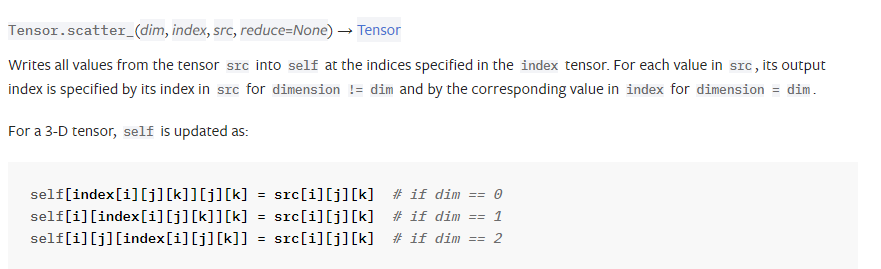我们可以看到 官网的第三个参数是src 也就是数据源，而这里是value 。。。真是奇怪。

对于tensor.scatter函数  可以看 这篇

对于torch.tensor.scatter（）这个函数的理解。_亮子李的博客-CSDN博客

相信大家对scatter 都有了理解。 我们回来。

这里先创建一个 长是512 *200 = 102400 宽是10的向量。

而sim_labels的大小是 （102400，1） 这个scatter做了什么呢 ？ 如下

one_hot = torch.tensor
([[0,0,0,0,0,0,0,0,0,0],
[0,0,0,0,0,0,0,0,0,0]])

sim_label = torch.tensor([,])
print(one_hot.scatter(-1,sim_label,value=1))
tensor([[0, 0, 0, 1, 0, 0, 0, 0, 0, 0],
[0, 0, 0, 0, 1, 0, 0, 0, 0, 0]])

也就是把每行标签对应得数字那一列变为1 ，如果这行特征得标签是1 就把第一个数变为1，这样子。  类似的有102400行。 得到onehot后 按我得想法，  就统计200行中哪一列的1最多呗。 比如前200行里 第3列的1对多， 就说明第一个样本最近的200个里，最多的标签是2 ，。我们看看他们怎么做的。

pred_scores = torch.sum(one_hot_label.view(feature.size(0), -1, classes) * sim_weight.unsqueeze(dim=-1), dim=1)
one_hot_label.view(feature.size(0), -1, classes)

这句可以理解。 变回512 *200*10 这样就可以统计各自的两百个了。

sim_weight.unsqueeze(dim=-1)

sim_weight 虽然在上面做了一点变换，但是我们其实不用管他，因为上面只是一种归一化的方式，我们依然可以把它看作最近 当前样本特征和两百个点特征的乘积。unsqueeze 表示扩充一维 在最后， sim_weight就变成了 512 *200 *1。 我们如何理解这个pred_score呢？ 我们不要看512个样本。 我们只看一个样本。 对于一个样本。他的one_label是200*10 而sim_weight就是200 *1  特征的点乘结果，也就是200个相似度分数 。 从两行 看两百行  很显然 就是让各行的标签1 乘上那个相似度分数。  之后再对200这个维度求和，就得到了各个标签相似的分数的和。 维度1*10

c = one_hot.scatter(-1,sim_label,value=1)
d = torch.tensor([,])
print(c*d)

#################
tensor([[0, 0, 0, 1, 0, 0, 0, 0, 0, 0],
[0, 0, 0, 0, 1, 0, 0, 0, 0, 0]])
tensor([[0, 0, 0, 3, 0, 0, 0, 0, 0, 0],
[0, 0, 0, 0, 4, 0, 0, 0, 0, 0]])

print(torch.sum(c*d,dim=0))

#########
tensor([0, 0, 0, 3, 4, 0, 0, 0, 0, 0])

看到这里我们明白了 。 这里的knn并不是简单的从众，他还要看影响力。 更相似的样本，他的标签对我们的结果的影响力更大。 这里相当于对标签做了一个加权求和。

回到512维 我们得到了512*10的矩阵 表示512个样本的各个标签的相似度分数 我们只要argsort就可以得到最大值的下标啦。 np.argsort这个函数可以对向量排序 然后返回他们原来的下标 des 表示可以降序。

    pred_labels = pred_scores.argsort(dim=-1, descending=True)
return pred_labels

得到标签 ， 回到原来的knn

            total_num += data.size(0)
total_top1 += (pred_labels[:, 0] == target).float().sum().item()
test_bar.set_postfix({'Accuracy':total_top1 / total_num * 100})
return total_top1 / total_num * 100

这里是计算top1  我估计如果计算top5 估计就是 target in labels[:,4]了 得到预测标签后准确率久很好算了。

# 5 ：线性验证

继续跟着主函数走 。 可以看到一堆保存的步骤。 然后进入linear_eval函数。  我猜测是用backbone抽特征然后直接预测结果的函数。


dataset=get_dataset(
transform=get_aug(train=False, train_classifier=True, **args.aug_kwargs),
train=True,
**args.dataset_kwargs
),
batch_size=args.eval.batch_size,
shuffle=True,
)
dataset=get_dataset(
transform=get_aug(train=False, train_classifier=False, **args.aug_kwargs),
train=False,
**args.dataset_kwargs
),
batch_size=args.eval.batch_size,
shuffle=False,
)

model = get_backbone(args.model.backbone)
classifier = nn.Linear(in_features=model.output_dim, out_features=10, bias=True).to(args.device)


先读取训练集和测试集， 然后 model是resnet  一个分类器是 一个全连接。 我好奇的是为什么不直接把backbone最后一层的恒等映射改为这个分类器呢 ？

msg = model.load_state_dict({k[9:]:v for k, v in save_dict['state_dict'].items() if k.startswith('backbone.')}, strict=True)

载入模型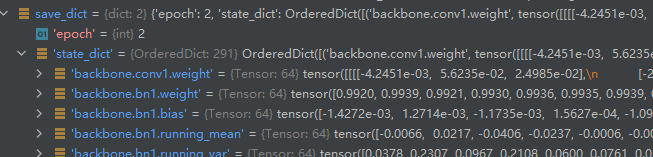k长这个样子  取出那些以backb开头的层 就是resnet的层。 然后去掉前面9个字母 就是resnet的名字。

    classifier = torch.nn.DataParallel(classifier)
# define optimizer
optimizer = get_optimizer(
args.eval.optimizer.name, classifier,
lr=args.eval.base_lr*args.eval.batch_size/256,
momentum=args.eval.optimizer.momentum,
weight_decay=args.eval.optimizer.weight_decay)

# define lr scheduler
lr_scheduler = LR_Scheduler(
optimizer,
args.eval.warmup_epochs, args.eval.warmup_lr*args.eval.batch_size/256,
args.eval.num_epochs, args.eval.base_lr*args.eval.batch_size/256, args.eval.final_lr*args.eval.batch_size/256,
)

loss_meter = AverageMeter(name='Loss')
acc_meter = AverageMeter(name='Accuracy')

定义优化器和loss 最下面这个averagemeter是啥呀

查了一下 就是一个类似于队列这种的 数据结构。 然后可以更新  关键是可以求平均。

    for epoch in global_progress:
loss_meter.reset()
model.eval()
classifier.train()
local_progress = tqdm(train_loader, desc=f'Epoch {epoch}/{args.eval.num_epochs}', disable=True)

for idx, (images, labels) in enumerate(local_progress):

feature = model(images.to(args.device))

preds = classifier(feature)

loss = F.cross_entropy(preds, labels.to(args.device))

loss.backward()
optimizer.step()
loss_meter.update(loss.item())
lr = lr_scheduler.step()
local_progress.set_postfix({'lr':lr, "loss":loss_meter.val, 'loss_avg':loss_meter.avg})


然后定义好后 就是一个普通的训练过程了 。 值得注意的是 model是eval模型 也就是他是冻住的，参数不改变。而classfier是可以改变的，  梯度回传也只回传分类头的梯度， 这里就只训练分类器。


classifier.eval()
correct, total = 0, 0
acc_meter.reset()
for idx, (images, labels) in enumerate(test_loader):
feature = model(images.to(args.device))
preds = classifier(feature).argmax(dim=1)
correct = (preds == labels.to(args.device)).sum().item()
acc_meter.update(correct/preds.shape)
print(f'Accuracy = {acc_meter.avg*100:.2f}')

普通的测试。

# 6 ： 用自己数据集进行对比学习。

路走远了，别忘了开始的方向。 我们是用对比学习解决食物分类的问题的。

我们要做的有几件事情。

## 第一：  改数据集 ：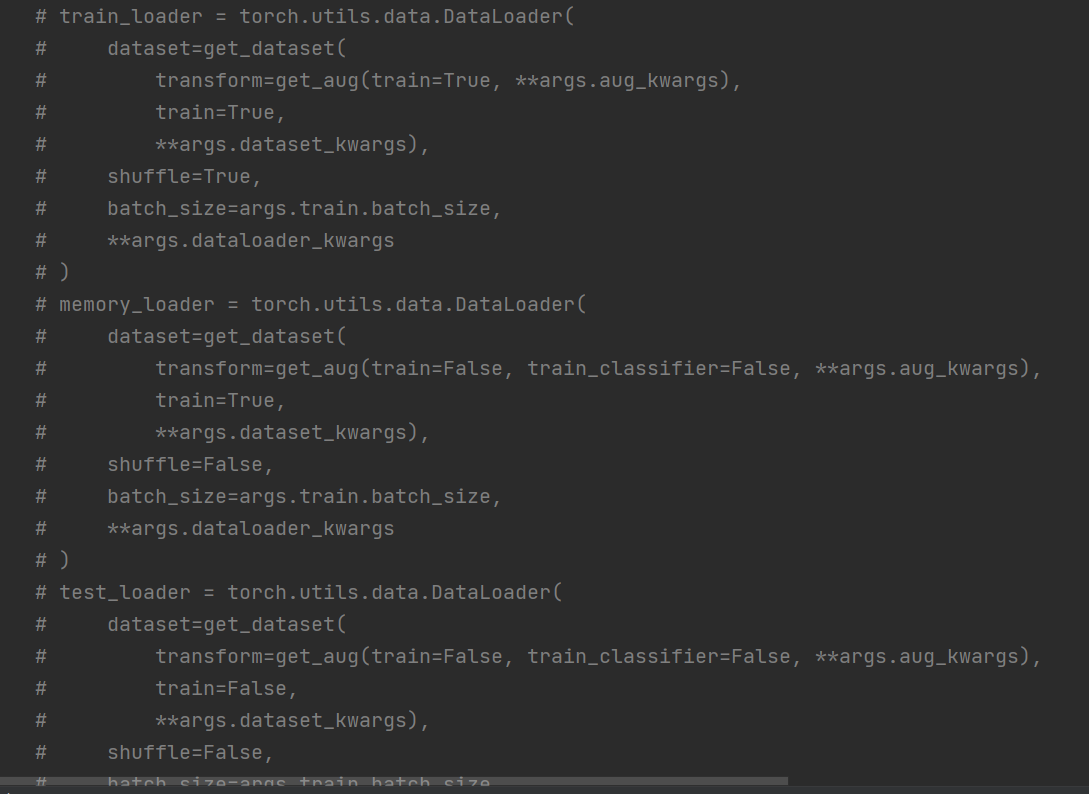把它原来的三个数据集全#了。

然后 加入自己的数据集。  使用他的增广方式。 但在增广前   需要在 dataset的get里加 topil 因为他的增广里没有这个。

hw3食物分类有三个数据集：

一个有标签训练集 我用来当memory

一个无标签训练集  我用来当train

一个验证集 我用来测试。

pil_trans = transforms.ToPILImage()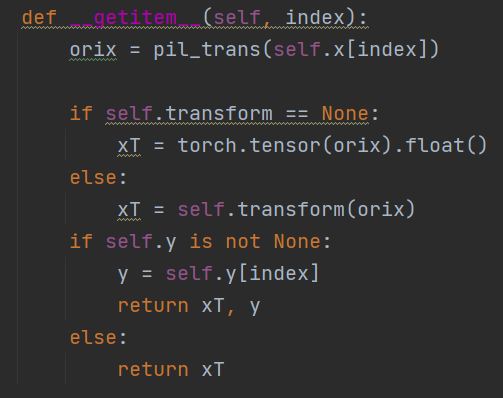filepath = '/home/lhy/hw3/food-11'
train_loader = getDataLoader(filepath, 'train_unl', True, args.train.batch_size, transform=get_aug(train=True, train_classifier=False, **args.aug_kwargs))
memory_loader = getDataLoader(filepath, 'train', False,args.train.batch_size, transform=get_aug(train=False, train_classifier=False, **args.aug_kwargs))
test_loader = getDataLoader(filepath, 'val', False,args.train.batch_size, transform=get_aug(train=False, train_classifier=False, **args.aug_kwargs))

# 2 改变batch_size和图片大小。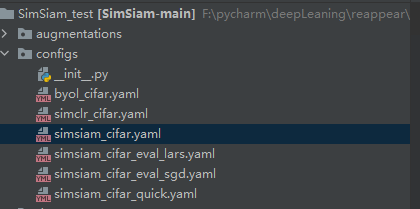在这个文件里改batch  你会发现这个对比学习的模型 出奇的占内存，当我图片大小为224时，我的batch只能设置为32.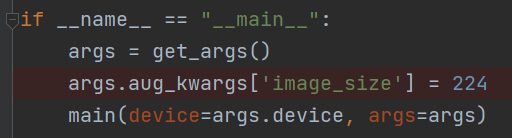main函数里改imagesize 为自己的。

点运行。O了 。 然后发现效果并不是很好，后面发现是准确率涨的太慢了。。。。。

监督学习 正确率能达到60 已经是极限了。 而无监督模型  我训练了大概60多个小时 ， 最后得到了71的准确率 。  我估计这个快到strong了都。 因为真的很难达到啊。 这说明，对比学习真的是有用的！！！ 令人震惊。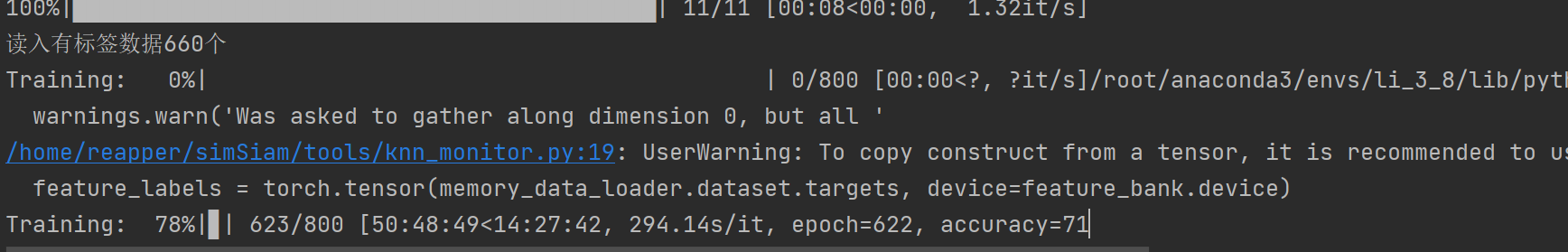又训练了100个epoch，准确率并没有上升，看来71已经几乎是极限了。

展开全文• Pytorch实现有监督对比学习损失函数关于对比损失Pytorch实现有监督对比损失END 关于对比损失 无监督对比损失，通常视数据增强后的图像与原图像互为正例。而对于有监督对比损失来说，可以将同一batch中标签相同的视为...

# 关于对比损失

无监督对比损失，通常视数据增强后的图像与原图像互为正例。而对于有监督对比损失来说，可以将同一batch中标签相同的视为正例，与它不同标签的视为负例。对比学习能够使得同类更近，不同类更远。有监督对比损失公式如下。

## 有监督对比损失数学公式## Pytorch实现有监督对比损失

话不多说，直接看代码。为了更好的说明有监督对比损失的整个实现过程，以下代码没有经过系统整理，从一个例子，一步一步地计算出损失。若是理解了每一步，那系统整理应该没什么问题。

import torch
import torch.nn.functional as F

T = 0.5  #温度参数T
label = torch.tensor([1,0,1,0,1])
n = label.shape  # batch

#假设我们的输入是5 * 3  5是batch，3是句向量
representations = torch.tensor([[1, 2, 3],[1.2, 2.2, 3.3],
[1.3, 2.3, 4.3],[1.5, 2.6, 3.9],
[5.1, 2.1, 3.4]])

#这步得到它的相似度矩阵
similarity_matrix = F.cosine_similarity(representations.unsqueeze(1), representations.unsqueeze(0), dim=2)
#这步得到它的label矩阵，相同label的位置为1
mask = torch.ones_like(similarity_matrix) * (label.expand(n, n).eq(label.expand(n, n).t()))

#这步得到它的不同类的矩阵，不同类的位置为1

#这步产生一个对角线全为0的，其他位置为1的矩阵
mask_dui_jiao_0 = torch.ones(n ,n) - torch.eye(n, n )

#这步给相似度矩阵求exp,并且除以温度参数T
similarity_matrix = torch.exp(similarity_matrix/T)

#这步将相似度矩阵的对角线上的值全置0，因为对比损失不需要自己与自己的相似度

#这步产生了相同类别的相似度矩阵，标签相同的位置保存它们的相似度，其他位置都是0，对角线上也为0

#用原先的对角线为0的相似度矩阵减去相同类别的相似度矩阵就是不同类别的相似度矩阵
no_sim = similarity_matrix - sim

#把不同类别的相似度矩阵按行求和，得到的是对比损失的分母(还差一个与分子相同的那个相似度，后面会加上)
no_sim_sum = torch.sum(no_sim , dim=1)

'''
将上面的矩阵扩展一下，再转置，加到sim（也就是相同标签的矩阵上），然后再把sim矩阵与sim_num矩阵做除法。
至于为什么这么做，就是因为对比损失的分母存在一个同类别的相似度，就是分子的数据。做了除法之后，就能得到
每个标签相同的相似度与它不同标签的相似度的值，它们在一个矩阵（loss矩阵）中。
'''
no_sim_sum_expend = no_sim_sum.repeat(n, 1).T
sim_sum  = sim + no_sim_sum_expend
loss = torch.div(sim , sim_sum)

'''
由于loss矩阵中，存在0数值，那么在求-log的时候会出错。这时候，我们就将loss矩阵里面为0的地方
全部加上1，然后再去求loss矩阵的值，那么-log1 = 0 ，就是我们想要的。
'''
loss = mask_no_sim + loss + torch.eye(n, n )

#接下来就是算一个批次中的loss了
loss = -torch.log(loss)  #求-log
loss = torch.sum(torch.sum(loss, dim=1) )/(2*n)  #将所有数据都加起来除以2n

print(loss)  #0.9821
#最后一步也可以写为---建议用这个， (len(torch.nonzero(loss)))表示一个批次中样本对个数的一半
loss = torch.sum(torch.sum(loss, dim=1)) / (len(torch.nonzero(loss)))


## END

大致实现过程就是这样，如果有什么问题可以随时提出。或者有什么更好的实现方法，也欢迎共享。若你要使用该损失发文章，请引用：
“Chen, L., Wang, F., Yang, R. et al. Representation learning from noisy user-tagged data for sentiment classification. Int. J. Mach. Learn. & Cyber. (2022). https://doi.org/10.1007/s13042-022-01622-7”

展开全文人工智能 神经网络 机器学习
• Generative Methods（生成式方法）这类方法以自编码器为代表，主要关注...与生成式学习比较，对比学习不需要关注实例上繁琐的细节，只需要在抽象语义级别的特征空间上学会对数据的区分即可，因此模型以及其优化...

# 1. 无监督学习分类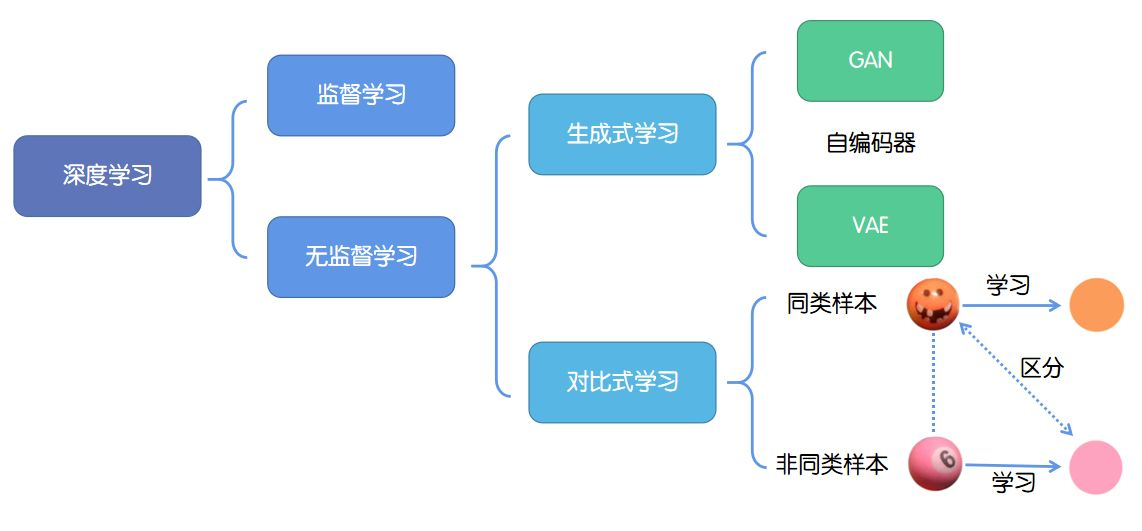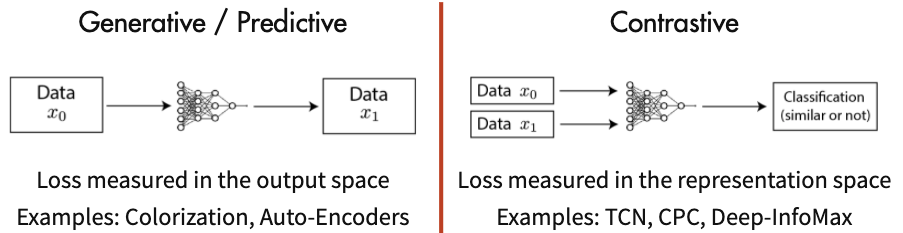• 生成式方法：以自编码器为代表，主要关注pixel label的loss。举例来说，在自编码器中对数据样本编码成特征再解码重构，这里认为重构的效果比较好则说明模型学到了比较好的特征表达，而重构的效果通过pixel label的loss来衡量。
• 对比式学习：着重于学习同类实例之间的共同特征，区分非同类实例之间的不同之处。不需要关注实例上繁琐的细节，只需要在抽象语义级别的特征空间上学会对数据的区分即可，因此模型以及其优化变得更加简单，且泛化能力更强。

# 2. 对比学习Contrastive Learning

## 2.1 介绍

对比学习（Contrastive Learning）是一种最近非常流行的自监督学习（Self-Supervised Learning）技术，它利用无标签训练数据生成的成对的增广数据（augmentations）去定义一个分类任务作为前置任务（pretext task），目的是学到一个足够好的深度表示（deep embedding）。

对比学习的前置任务则是将每一个实例（instance）作为一个类别（class），然后去学习一个不变的实例表示（invariant instance representation）。具体实现方式为，首先为每个实例生成一对样本，然后将它们输入一个编码器（encoder），然后利用对比损失（contrastive loss）去训练这个编码器。这个对比损失会鼓励，由同一个实例产生的正样本对的表示（embeddings）互相靠近，而由不同实例产生的负样本对的表示互相远离。

对比学习的目标是学习一个编码器，此编码器对同类数据进行相似的编码，并使不同类的数据的编码结果尽可能的不同

## 2.2 正负样本选择（单个正样本+多个负样本）

Contrastive Methods主要的难点在于如何构造正负样本。

### Contrastive Predictive Coding （CPC）

对于语音和文本，可以充分利用了不同的 k 时间步长来采集正样本，而负样本可以从序列随机取样来得到。对于图像任务，可以使用 pixelCNN 的方式将其转化成一个序列类型具体的：给定声音序列上下文Ct和Xt，我们推断预测Xt+1位置上的声音信号。此时，正样本Xt+1，负样本随机采样Xt*

### Deep InfoMax

正样本就是图片的 global feature 和中间 feature map 上个的 local feature，而负样本就是另外一张图片

### Contrastive MultiView Coding

除了像上面这样去构建正负样本，还可以通过多模态的信息去构造。比如同一张图片的 RGB图 和 深度图去选择正样本，而且通过这个方式，每个 anchor 不仅仅只有一个正样本，可以通过多模态得到多个正样本。但是如何节省内存和空间获得大量的负样本仍然没有很好地解决。

### SimCLR

如果一个批包含N个图像，那么对于每个图像，我们将得到2个表示，这总共占2*N个表示。对于一个特定的表示x，有一个表示与x形成正对（与x来自同一个图像的表示，通过旋转、裁剪、剪切、噪声、模糊、Sobel滤波等数据增强方式），其余所有表示（正好是2*N–2）与x形成负对

### Supervised Contrastive Learning

有标签数据集，除了同一图像不同表示作为正样本，同类别的数据也作为正样本；不同类别的数据作为负样本

## 2.3 损失函数Loss

Contrastive Loss: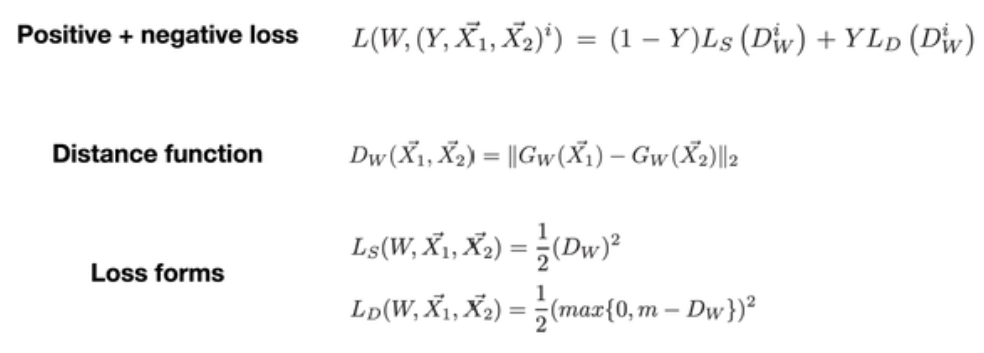W 是网络权重；Y是成对标签，如果X1，X2这对样本属于同一个类，Y=0，属于不同类则 Y=1。Dw 是 X1 与 X2 在潜变量空间的欧几里德距离。

InfoNCE Loss表示为：    (增加了更多的不相似样本)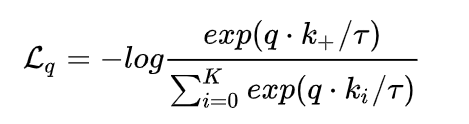Supervised Contrastive Learning Loss: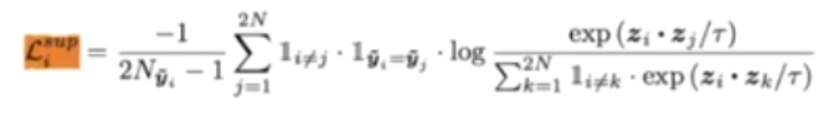具象化表示：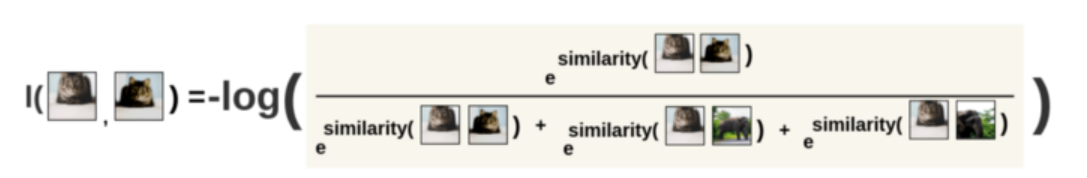1） 计算相似度

2） Cross-Entropy

## 2.4 代码实现Loss

import torch
import torch.nn as nn

def  similarity(q,k,queue):
T=0.07
# compute logits
# Einstein sum is more intuitive;相似度计算
# positive logits: Nx1
l_pos = torch.einsum('nc,nc->n', [q, k]).unsqueeze(-1)
# negative logits: NxK
l_neg = torch.einsum('nc,ck->nk', [q, queue])

# 正负样本拼接
# logits: Nx(1+K)
logits = torch.cat([l_pos, l_neg], dim=1)

# apply temperature  超参数
logits /= T

return logits

batch=32
N=15
im_q=torch.randn(batch,12)
im_k=torch.rand(batch,12)
im_neg=torch.rand(12,N)   #队列中取N个作为负样本

# define loss function (criterion) and optimizer
criterion = nn.CrossEntropyLoss()
# compute output
output = similarity(im_q, im_k,im_neg)
# labels: positive key indicators  生成标签
labels = torch.zeros(output.shape, dtype=torch.long)

loss = criterion(output, labels)


reference：

https://zhuanlan.zhihu.com/p/130914340

https://zhuanlan.zhihu.com/p/346686467

展开全文自监督学习 pytorch代码
• 本文主要分享一下Google今年提出的《Self-supervised Learning for Large-scale Item Recommendations》如何采用对比学习解决推荐长尾问题 。一、 定义长尾效应：20%的热门item占据了80%的曝光量，剩下80%的...计算机视觉 人工智能
• 对比学习的思想起源于无监督学习，相比于监督学习算法，无监督学习由于没有标签的指导，训练过程学习样本的特征会更加困难。对比学习的核心思想就是通过数据增强构造原来样本的多样性，损失函数的机器学习
• ©作者 |侯宇蓬单位 |中国人民大学文章来源 |RUC AI Box随着对比学习（Contrastive Learning）在 CV、NLP 等领域大放异彩，其研究热度近年来也逐步走...大数据 python 神经网络 机器学习
• 代码： import difflib import sys def readfile(filename): try: fileHandle = open(filename, 'r+') text = fileHandle.read().splitlines() fileHandle.close() return text except IOError as error:...python
• 对比学习 ——simsiam 代码解析。.doc
• 基于自监督对比学习的深度神经网络对抗鲁棒性提升.pdf
• 代码可以运行，是关于LMS和RLS算法的对比仿真实验，分别有在不同信噪比和不同步长下，学习曲线的对比图。
• 机器学习入门项目，kaggle里的项目房价预测，适合初学者学习数据分析及机器学习算法，入门Kaggle.
• 对比学习的核心思想是吸引正样本对，排斥负样本对。对比学习在无监督（自监督）表征学习中广泛应用。基于孪生网络的简单高效的对比学习实例方法已经被开发出来。实际上，对比学习方法从大量的负样本的获益，InfoDist...SimSiam 机器学习
• ## 对比学习火了

千次阅读 2021-06-15 18:13:11
前言 最近对比学习火起来了，其思想特别简单但有效，总结起来就是： 对一条样本x1通过数据增强得到x2，那么这就是一对正样本对，和其他样本就是负样本对。自然语言处理 机器学习
•   最近在基于对比学习做实验，github有许多实现，虽然直接套用即可，但是细看之下，损失函数部分甚是疑惑，故学习并记录于此。关于对比学习的内容网络上已经有很多内容了，因此不再赘述。本文重在对InfoNCE的两种...
• 本文粗略介绍了对比学习的基本原理以及常见方法。 什么是对比学习对比学习是一种自监督或者无监督学习的一种方法。通过对比未知样本和正负样本的相近程度，来给未知样本进行正负归类。 最早运用于判定图像表征...机器学习 计算机视觉
• 目录对比学习(Contrastive Learning)Gelato Bet （冰淇淋赌注）与何凯明组的MoCo模型监督学习vs自监督学习生成式学习与对比学习对比学习的工作方式对比学习范例从DIM(Deep InfoMax)到DGI(Deep Graph InfoMax)Deep ...图嵌入 机器学习 人工智能
• 1、图像批量处理-对比度 from skimage import data_dir, exposure,io, transform, color import numpy as np def convert_gray(f): rgb = io.imread(f) # 依次读取rgb图片 gamma = exposure.adjust_gamma(rgb, 3...python 计算机视觉 opencv
• 自监督学习在CV和NLP已经用的很多了，那很自然也会被迅速引进并占坑到推荐系统领域咯。而发掘推荐数据上的自监督信号，其实也是十分有利于推荐系统的，主要有以下优势： 舒缓数据稀疏。一般来说推荐系统的数据集，...
• - 传统的对比学习是data-level的，本文改进了FedAvg的本地模型训练阶段，提出了model-level的联邦对比学习（Model-Contrastive Federated Learning） - 作者从NT-Xent loss中获得灵感，提出了model-contrastive loss...联邦学习
• 目录0，参考文献和前置知识和阅读注意1，[ECCV20]Contrastive Learning for Unpaired Image-to-Image Translation1.1，创新点和架构1.2，multi-layer、patchwise的对比学习 0，参考文献和前置知识和阅读注意 参考...计算机视觉
•tensorflow
• 在进行神经网络反复训练试验后发现，每次的训练结果都有微小的波动，根据查阅相关资料，总结了神经网络模型代码复现的主要注意的几点： 首先强调，自己用的试验平台是PyTorch 1.9 GPU版本，CUDA为10.2版本，显卡为...神经网络 pytorch 代码规范
• 我想大家将对比学习与推荐系统结合主要有以下四个原因：一、是因为数据的稀疏性。众所周知，在推荐系统中有点击的数据是非常少的，可能系统推荐了十篇文章，用户只点击了一篇文章，因此我们可以通过自监督学习对点击...
• 点击下方卡片，关注“CVer”公众号AI/CV重磅干货，第一时间送达作者丨小马转载自丨极市平台导读作者专为目标检测任务“量身定制”了对比学习框架DetCo，在PASCAL VOC数据集上...计算机视觉 机器学习 人工智能 编程语言
• 在深度学习中，优化器是其重要组成部分，本文来介绍一下常用优化器（SGD, Momentum, Nesterov Momentum, AdaGrad, RMS Prop, Adam）的伪代码并对他们进行对比。 1. SGD SGD的伪代码如下： SGD的梯度更新公式...机器学习 人工智能...